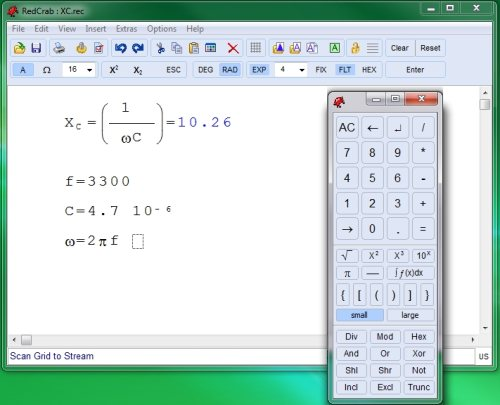Editor Ratings:
User Ratings:
[Total: 0 Average: 0]

RedCrab is a free scientific calculator software that lets you perform complex mathematical calculations. Unlike other free scientific calculator software, what sets RedCrab apart is its nice big interface that make it very easy to enter all type of complex formulas and equations, and use results of a formula in subsequent calculations. Apart from that, this scientific calculator software also lets you import images in the formula editor section so that you can easily explain to anyone what is going on.

This scientific calculator freeware supports almost all type of mathematical calculations, like, Square root, log, power, pi, Div, Mod, Hex, trignometric functions, f(x), and more.To enter any formula, you just pick the corresponding function from its toolbox, and start building formula. This scientific calculator freeware also lets you save formulas to create your own formula library, and easily reference them in other more complicated formulas.

RedCrab is a nice scientific calculator software that is completely free, and easy to use. Download RedCrab scientific calculator freeware.